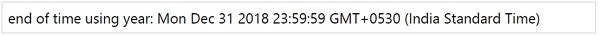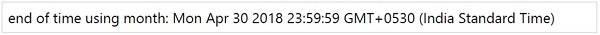# MomentJS - End of Time

End of Time method will set the display to the end of the unit given.

## Syntax

```moment().endtOf(String);
```

## Example using year as the input

```var day = moment().endOf('year');
```

This will display the last day of the year and will set the time to 23:59:59 as shown below −## Example using month as the input

```var day = moment().endOf('month');
```

This will display the last day of the month and will set the time ie hours, minutes and seconds to 23:59:59 as shown below −momentjs_manipulate_date_and_time.htm